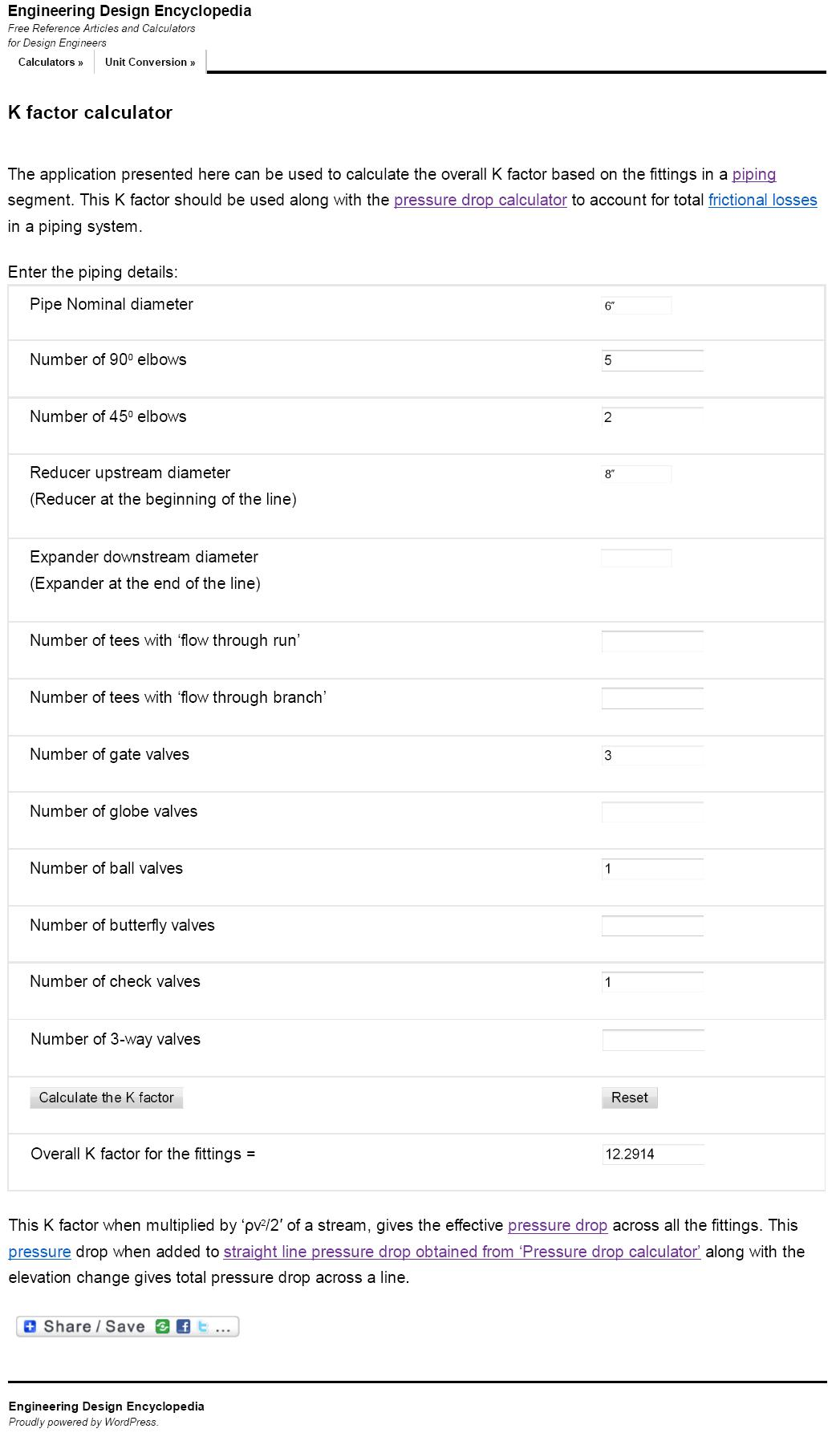# Equivalent Length of Pipe Fittings

The equivalent length of pipe fittings refers to the length of straight pipe that would create the same pressure drop as the fitting under consideration. This concept is important in fluid mechanics and is used in the calculation of pressure drop in piping systems. By using the concept of equivalent length, engineers can design piping systems that minimize pressure drop and optimize the flow of fluids through the system.

## Equivalent length of pipe fittings

The Equivalent Length of a pipe fitting is defined as the length of pipe with the same size as the fitting that would create the same pressure drop as the fitting. Research has shown that the Equivalent Length (Le) is greater for larger fittings of the same type (e.g., long radius elbow). However, dividing Le by the inside diameter of the pipe (D) results in a constant ratio (Le/D) for the fitting type.

This approach has two benefits: it significantly reduces the amount of required information and eliminates the issue of using various units to measure lengths and diameters, as the ratio Le/D has no dimensions. As a result, the data tables provided in this article can be used with any unit system, provided that D and Le are measured in the same units.

Pipe fittings, such as elbows, tees, valves, and reducers, cause changes in the direction and velocity of the fluid flow, which leads to pressure drop. The pressure drop caused by a fitting is a function of its geometry and the flow rate. The roughness of the pipe also has an impact on the pressure drop.

To simplify calculations, engineers use the concept of equivalent length, which allows them to model the pressure drop caused by fittings as an equivalent length of straight pipe. The equivalent length of a fitting is determined by experiment or by using empirical formulas. The total equivalent length of a piping system is the sum of the equivalent lengths of all the fittings and the straight pipe sections.

## Equivalent Length Calculation for Pipe Fittings

This tutorial uses sample calculations to demonstrate how to calculate equivalent length for piping fittings, joints and valves.

#### Problem Statement

Calculate the equivalent length and combined K-factor for following set of fittings,900 elbows - 5 nos. 450 elbows - 2 nos.Gate valves - 3 nos.Ball valve - 1 no.Check valve - 1 no. The line size is 6 inches while there is a 8"-6" reducer at the beginning of the line.

The 6" line with 'STD' schedule handles 100,000 kg/hr of water. Approximate length of this line is around 200m. This water stream is available at 5 barg pressure and 300C.

#### Solution

This sample problem is solved in following 3 basic steps.

###### Step 1.

First step of solving this sample problem requires determination the important physical properties of given fluid (water) at given temperature and pressure conditions.

Liquid density: We need to first determine density of water at 300C. The equation for density is ρ=m/v. If you know density ρr at some temperature Tr, there is a following formula for density: ρ=ρr[1+b(T−Tr)], where ρ is the density at temperature T and b is called coefficient of cubical expansion, evaluated at reference temperature and density (ρr and Tr).

We can calculate easily it using EnggCyclopedia's Liquid Density Calculator, water density at 300C =993.41 kg/m3

Liquid viscosity: We require value of viscosity of water at particular temperature for pump sizing calculation. We can calculate it  using EnggCyclopedia's Liquid Viscosity Calculator, water viscosity at 300C =0.81 cP

From EnggCyclopedia's standard pipe dimensions calculator, for 6" pipe with STD schedule, internal diameter is 154.1 mm.

###### Step 2.

Total K factor for the set of fittings can be calculated using EnggCyclopedia's K factor calculator. Refer to the screenshot below for demonstration of use of this calculator.###### Step 3.

In the third step of solving this sample problem, inputs from steps 1 and 2 are fed to EnggCylopedia's equivalent length calculator to get equivalent length for the given set of fittings. Refer to the screenshot given below for guidance to use the calculator.Thus, the set of fittings in given 6" line are equivalent to 60.36 m of that line. Along with the original 200 m of straight pipe, the total equivalent length of the given 6" line becomes 260.36 m.

This length can be used to quickly calculate variation of pressure drop for minor variations in the flowrate, using EnggCyclopedia's pipe pressure drop calculator. For major variations in the flowrate, the Darcy friction factor and hence the equivalent length has to be recalculated.